Courses

# Electrical & Electronic Measurements - MCQ Test 1

## 10 Questions MCQ Test GATE Electrical Engineering (EE) 2022 Mock Test Series | Electrical & Electronic Measurements - MCQ Test 1

Description
This mock test of Electrical & Electronic Measurements - MCQ Test 1 for GATE helps you for every GATE entrance exam. This contains 10 Multiple Choice Questions for GATE Electrical & Electronic Measurements - MCQ Test 1 (mcq) to study with solutions a complete question bank. The solved questions answers in this Electrical & Electronic Measurements - MCQ Test 1 quiz give you a good mix of easy questions and tough questions. GATE students definitely take this Electrical & Electronic Measurements - MCQ Test 1 exercise for a better result in the exam. You can find other Electrical & Electronic Measurements - MCQ Test 1 extra questions, long questions & short questions for GATE on EduRev as well by searching above.
QUESTION: 1

Solution:
QUESTION: 2

### Match List-I (Bridge) with List-II (Parameter to be measured) and select the correct answer: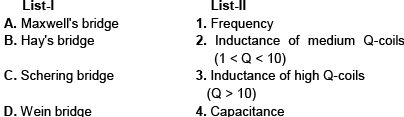Codes: A B C D

Solution:

• The Wein's bridge is primarily known as a frequency determining bridge. It may be employed in a harmonic distortion analyzer, where it is used as notch filter, discriminating against one specific frequency. The Wein's bridge also finds applications in audio and HF oscillators as the frequency determining device.

• The Hay's bridge is suited for the measurement of high Q inductors, especially those inductors having a Q greater than 10. For inductors having Q values smaller than 10, the term (1/Q)2 in the expression for inductance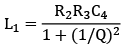becomes rather important and thus cannot be neglected. Hence this bridge is not suited for measurement of coils having Q less than 10 and for these applications a Maxwell's bridge is more suited.

QUESTION: 3

### Which of the following are the characteristics of a thermocouple type of indicating instrument? 1. Its accuracy is very high, as high as about 1 percent 2. It has linear scale because a d'Arsonval movement is used for measuring the output. 3. It is a RF instrument and can be used for frequency up to about 50 MHz. 4. It cannot be damaged by overloads

Solution:

• Thermo-couple instrument has a "squarelaw response" so it does not have a linear scale.

• Their accuracy can be as high as 1% for frequencies upto 50 MHz and can be classified as RF instruments. Above this frequency the effective resistance of heater wire is increased on account of skin effect, thereby reducing the accuracy. Tubular designs are used for heater wire above 3A to reduce errors on account of skin effect produced by high frequencies. For small currents upto 3A, the heater wire is solid and very thin.

• At normal rated current, the heater attains a temperature of 300 °C. If we pass twice the rated current, the heater would give a temperature of nearly 4 times the normal temperature i.e., 1200 °C. It is obvious that square law rate will bring the heater to nearly its burn out temperature. Thus it is imperative that the heater be protected against damaging over loads. The fuses do not provide any protection as due to overload the heater wire may burn out before fuse blows out. Thus the overload capacity of thermo-couple instruments is small as compared with other instruments and is about 150 percent of the full scale current.

QUESTION: 4

Consider the following equations which can be derived from the ac bridges shown in the Figure 1 and Figure 2 by assuming ∆L/L = 0.1 and R = ωL :

1. V o1 = V o2

2. V o1 = 0.05 V s

3. V o1 = 0.1 V s

4. V o2 = 0.05 V s

5. V o2 = 0.1 V s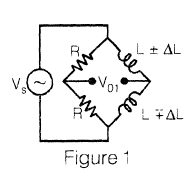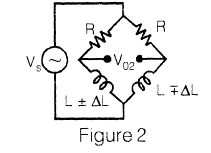The correct derived equations from these figures of ac bridges are

Solution: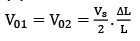on solving bridge circuit.

QUESTION: 5

In a single-phase power factor meter the controlling torque is

Solution:

There will be two deflecting torques, one acting on coil A and the other on coil B. The coil windings are so arranged that the torques due to the two coils are opposite in direction. So, there is no controlling torque.

QUESTION: 6

A dc circuit can be represented by an internal voltage source of 50V with an output resistance of 100 kΩ. In order to achieve accuracy better than 99% for voltage measurement across its terminals, the voltage measuring device should have a resistance of at least

Solution: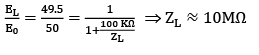QUESTION: 7

In electrodynamometer type wattmeters, the inductance of pressure coil produces error. The error is

Solution:

The error caused by pressure coil inductance is VI sin ϕ tan β. W ith low power factor, the value of ϕ is large and therefore, the error is correspondingly large.

QUESTION: 8

An ac voltmeter using full-wave rectification and having a sinusoidal input has ac sensitivity equal to

Solution:

For full-wave rectifier type of instruments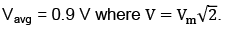QUESTION: 9

Time division multiplexing is used when the data to be transmitted is

Solution:
QUESTION: 10

If an induction type energy meter runs fast, it can be slowed down by

Solution:

Braking torque TD ∝ R2 where R is the radial position of braking magnet with respect to the centre of the disc.